# Degree of a mapping

(diff) ← Older revision | Latest revision (diff) | Newer revision → (diff)

degree of a continuous mapping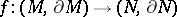between connected compact manifolds of identical dimension

An integer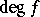such that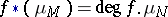, where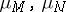are the fundamental classes (cf. Fundamental class) of the manifoldsandover the ring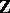or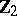, and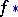is the induced mapping. In the case of non-orientable manifolds, the degree of the mapping is uniquely defined modulo 2. If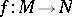is a differentiable mapping between closed differentiable manifolds, thenmodulo 2 coincides with the number of inverse images of a regular valueof. In the case of oriented manifolds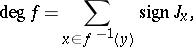where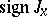is the sign of the Jacobian ofat a point(the Browder degree).

For a continuous mapping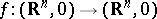and an isolated pointin the inverse image of zero, the concept of the local degreeat the pointis defined: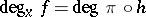, whereis the restriction ofonto a small sphere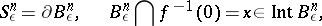andis the projection from zero onto the unit sphere. In the case of a differentiable, the formula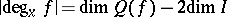holds, where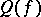is the ring of germs (cf. Germ) of smooth functions at zero, factorized by the ideal generated by the components of, andis the maximal ideal of the quotient ring relative to the property. Letbe the class of the Jacobian of the mapping. Then for a linear form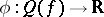such thatthe formula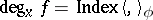holds, whereis a symmetric bilinear form on.

How to Cite This Entry:
Degree of a mapping. Encyclopedia of Mathematics. URL: http://encyclopediaofmath.org/index.php?title=Degree_of_a_mapping&oldid=14689
This article was adapted from an original article by A.V. Khokhlov (originator), which appeared in Encyclopedia of Mathematics - ISBN 1402006098. See original article